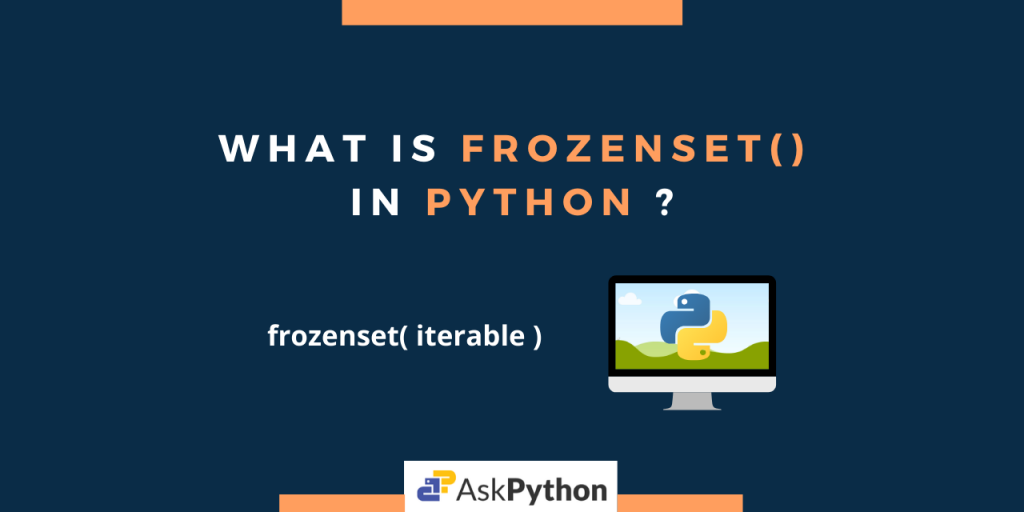# Python frozenset() – Every You Need To KnowHey there! So today we are here to discuss the Python frozenset() method.

So before we get into the method, let us know what a frozenset is.

## What is a frozenset?

A `frozenset` is an unordered, un-indexed, and immutable collection of elements. It provides all the functionalities that a set offers in Python, the only difference being the fact that a frozenset is immutable, i.e. can’t be changed after it is created.

Hence in simple words, frozen sets are immutable sets.

## The Python frozenset() Method

The Python `frozenset()` method returns a new frozenset object whose elements are taken from the passed `iterable`. If `iterable` is not specified, a new empty set is returned.

Note: The elements must be hashable.

```fz = frozenset([iterable])
```

When nothing is specified, the `frozenset()` method returns an empty frozenset object to `fz`.

To get a better understanding of how the method works, let us look at an example.

```# List Initialisation
list1 = [0, 1, 2, 3, 4, 5, 6]

fz = frozenset(list1)
print(fz)

fz = frozenset()  # empty frozenset
print(fz)

print("Type of fz: ", type(fz))
```

Output:

```frozenset({0, 1, 2, 3, 4, 5, 6})
frozenset()
Type of fz:  <class 'frozenset'>
```

Here, firstly we have initialized a list(`list1`) and then passed it as `iterable` to the `frozenset()` method. In return we get a frozenset object(fz) with elements from the list. When nothing is passed, fz is now an empty frozenset object.

## Frozenset Initialization

In the below-given example, we have initialized a frozenset using the Python `frozenset()` method by passing different iterables like list, tuple, set, and dictionary.

```# Initialisation
list1 = [1, 2, 3, 4, 5]

fz = frozenset(list1)  # from list object
print(fz)

fz = frozenset([1, 2, 3, 4, 5])  # from list
print(fz)

fz = frozenset({5: 'a', 4: 'B', 3: 'C', 2: 'D', 1: 'E', 0: '0'})# from dict
print(fz)

fz = frozenset({'Python', 'Java', 'C', 'C++', 'Kotlin', 'R'})# from set
print(fz)

fz = frozenset((17, 55, 26, 90, 75, 34)) # from tuple
print(fz)
```

Output:

```frozenset({1, 2, 3, 4, 5})
frozenset({1, 2, 3, 4, 5})
frozenset({0, 1, 2, 3, 4, 5})
frozenset({'Java', 'Kotlin', 'Python', 'C', 'R', 'C++'})
frozenset({34, 90, 75, 17, 55, 26})
```

For each case, we get a frozenset object with the corresponding iterable elements in it. But carefully note, that in the case of a dictionary only the keys are being considered.

## Operations on a Python frozenset

We can get names of all the methods related to a frozenset object using the dir() method.

```fo = frozenset([1, 2, 3, 4, 5])

print(dir(fo))
```

Output:

```['__and__', '__class__', '__contains__', '__delattr__', '__dir__', '__doc__', '__eq__', '__format__', '__ge__', '__getattribute__', '__gt__', '__hash__', '__init__', '__init_subclass__', '__iter__', '__le__', '__len__', '__lt__', '__ne__', '__new__', '__or__', '__rand__', '__reduce__', '__reduce_ex__', '__repr__', '__ror__', '__rsub__', '__rxor__', '__setattr__', '__sizeof__', '__str__', '__sub__', '__subclasshook__', '__xor__', 'copy', 'difference', 'intersection', 'isdisjoint', 'issubset', 'issuperset', 'symmetric_difference', 'union']
```

Notice from the above output, that various functions like add(), remove(), update(), pop() etc.(used to change/update elements, available for sets) are missing. Again this is due to the fact that a frozen set is immutable.

So now let us look at the available methods for frozen sets, using which we can perform various operations.

```fs = frozenset([1, 12, 23, 45, 67, 89, 100])

print("Given Frozenset =", fs)

fs_len = len(fs)
print("Length of Frozenset =", fs_len)

print("23 in fs? ", 23 in fs)

print("23 not in fs? ", 23 not in fs)

print("Sets are disjoint? ", fs.isdisjoint(frozenset([10, 5])))

print("Set is Subset? ", fs.issubset(set([1, 2, 3, 4, 12, 23, 45, 67, 89, 100])))

print("fs is superset? ", fs.issuperset(frozenset({1, 100})))

print("Union of sets: ", fs.union(frozenset([-1, -12])))

print("Intersection: ", fs.intersection(set([1, 10, 100])))

print("Difference: ", fs.difference(frozenset([1, 10, 100])))

print("Symmetric difference: ", fs.symmetric_difference(frozenset([1, 10, 100])))

fs_copy = fs.copy()
print("Copy of fs: ", fs_copy)
```

Output:

```Given Frozenset = frozenset({1, 67, 100, 12, 45, 23, 89})
Length of Frozenset = 7
23 in fs?  True
23 not in fs?  False
Sets are disjoint?  True
Set is Subset?  True
fs is superset?  True
Union of sets:  frozenset({1, 67, 100, 12, 45, -12, 23, 89, -1})
Intersection:  frozenset({1, 100})
Difference:  frozenset({67, 12, 45, 23, 89})
Symmetric difference:  frozenset({67, 10, 12, 45, 23, 89})
Copy of fs:  frozenset({1, 67, 100, 12, 45, 23, 89})
```

Here,

• len(s): Returns the length of the frozenset s,
• x in s: Checks whether x is present in frozenset s or not,
• x not in s: Returns True if x is not an element of frozenset s. Or else returns False,
• isdisjoint(other): Returns True if the set has no elements in common with `other`. Sets are disjoint if and only if their intersection is the empty set.
• issubset(other): Checks whether other contains elements of the frozenset or not,
• issuperset(other): Checks whether the frozenset contains elements of `other` or not,
• union(*others): Returns a frozenset containing the union of the provided other sets,
• intersection(*others): Returns a frozenset, with elements common to fs and all others passed,
• difference(*others): Returns a new frozenset with elements in the frozenset(fs) that are not in the others,
• symmetric_difference(other): Returns a new frozenset with elements in either fs or other but not both.

## Summing Up

That’s it for today. Hope you had a clear understanding of the Python `frozenset()` method.